# Data Structure and Algorithms - Shell Sort

#### Big Data Hadoop

Best Seller

89 Lectures 11.5 hours

#### Practical Data Science using Python

22 Lectures 6 hours

#### Data Science and Data Analysis with Python

50 Lectures 3.5 hours

Shell sort is a highly efficient sorting algorithm and is based on insertion sort algorithm. This algorithm avoids large shifts as in case of insertion sort, if the smaller value is to the far right and has to be moved to the far left.

This algorithm uses insertion sort on a widely spread elements, first to sort them and then sorts the less widely spaced elements. This spacing is termed as interval. This interval is calculated based on Knuth's formula as −

### Knuth's Formula

```h = h * 3 + 1
where −
h is interval with initial value 1
```

This algorithm is quite efficient for medium-sized data sets as its average and worst-case complexity of this algorithm depends on the gap sequence the best known is Ο(n), where n is the number of items. And the worst case space complexity is O(n).

## How Shell Sort Works?

Let us consider the following example to have an idea of how shell sort works. We take the same array we have used in our previous examples. For our example and ease of understanding, we take the interval of 4. Make a virtual sub-list of all values located at the interval of 4 positions. Here these values are {35, 14}, {33, 19}, {42, 27} and {10, 44}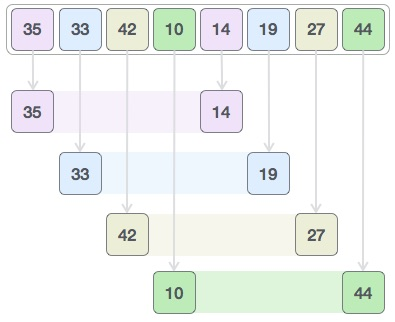We compare values in each sub-list and swap them (if necessary) in the original array. After this step, the new array should look like this −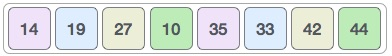Then, we take interval of 1 and this gap generates two sub-lists - {14, 27, 35, 42}, {19, 10, 33, 44}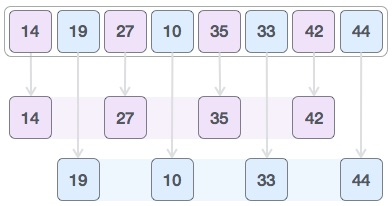We compare and swap the values, if required, in the original array. After this step, the array should look like this −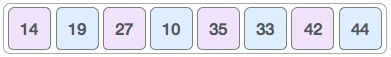Finally, we sort the rest of the array using interval of value 1. Shell sort uses insertion sort to sort the array.

Following is the step-by-step depiction −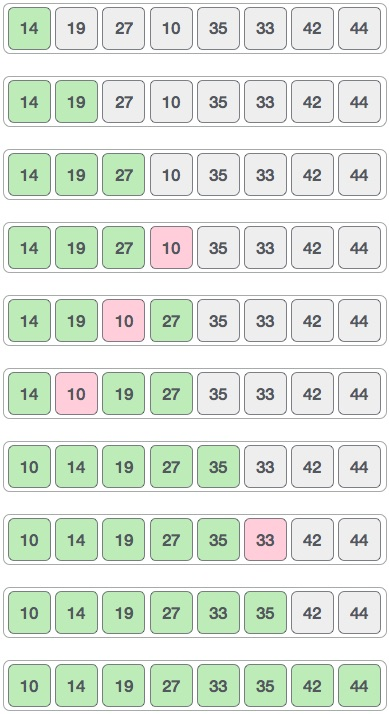We see that it required only four swaps to sort the rest of the array.

### Algorithm

Following is the algorithm for shell sort.

```Step 1 − Initialize the value of h
Step 2 − Divide the list into smaller sub-list of equal interval h
Step 3 − Sort these sub-lists using insertion sort
Step 3 − Repeat until complete list is sorted
```

## Pseudocode

Following is the pseudocode for shell sort.

```procedure shellSort()
A : array of items

/* calculate interval*/
while interval < A.length /3 do:
interval = interval * 3 + 1
end while

while interval > 0 do:

for outer = interval; outer < A.length; outer ++ do:

/* select value to be inserted */
valueToInsert = A[outer]
inner = outer;

/*shift element towards right*/
while inner > interval -1 && A[inner - interval] >= valueToInsert do:
A[inner] = A[inner - interval]
inner = inner - interval
end while

/* insert the number at hole position */
A[inner] = valueToInsert

end for

/* calculate interval*/
interval = (interval -1) /3;

end while

end procedure
```